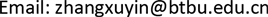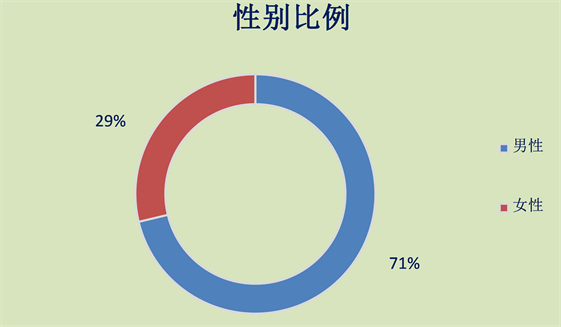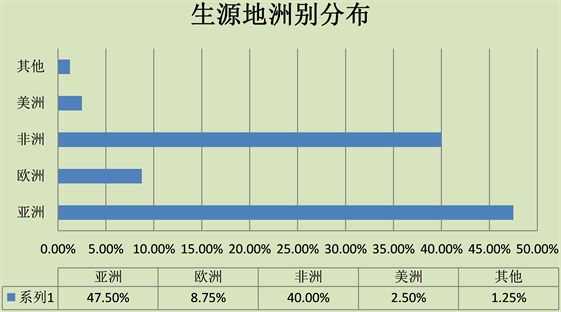﻿ 北京高校来华留学生学习成绩影响因素研究——以北京市为例 How to Evaluate Learning Performance of Overseas Student?—An Empritical Evidence from Beijing

Statistics and Application
Vol.07 No.04(2018), Article ID:26648,7 pages
10.12677/SA.2018.74055

How to Evaluate Learning Performance of Overseas Student?

—An Empritical Evidence from Beijing

Xuyin Zhang

Beijing Technology and Business University, BeijingReceived: Aug. 9th, 2018; accepted: Aug. 24th, 2018; published: Aug. 29th, 2018ABSTRACT

This research estimated influence factors of study result for overseas students. Hierarchical Linear Model was employed to analyze overseas students’ study achievements. Interview survey has conducted to collect primary data and has estimated the respondents by random coefficient model with binomial distribution in 3 typical universities. It indicated that positive motivation would promote overseas students to enhance their learning performance. The population effect and socioeconomic status significantly influenced overseas students’ learning behaviors. In conclusion the educational quality of overseas students would be accelerating by rasing up of scholarship coverage. And Chinese language training for overseas students should be promoted.

Keywords:Overseas Students, Learning Performance, HML

——以北京市为例1. 问题提出

2. 数据描述性统计

3. 研究方法与模型Figure 1. Gender ratioFigure 2. Distribution areas of student source

LEVEL1 MODEL

$\text{GRADE}={\beta }_{0}+{\beta }_{1}\left(\text{ACL}\right)+{\beta }_{2}\left(\text{SFI}\right)$ $+{\beta }_{3}\left(\text{GENDER}\right)+{\beta }_{4}\left(\text{EDUF}\right)+{\beta }_{5}\left(\text{EDUM}\right)+{\beta }_{6}\left(\text{DOM}\right)+{\beta }_{6}\left(\text{PJOB}\right)+r$

LEVEL2 MODEL

${\beta }_{0}={\gamma }_{00}+{\gamma }_{01}\left(\text{GDPINPC}\right)+{u}_{0}$

${\beta }_{1}={\gamma }_{1},\cdots ,{\beta }_{7}={\gamma }_{7}$

MIXED MODEL

$\begin{array}{l}\eta ={\gamma }_{00}+{\gamma }_{01}\left(\text{GDPINPC}\right)+{\gamma }_{10}\left(\text{ACL}\right)+{\gamma }_{20}\left(\text{SFI}\right)+{\gamma }_{30}\left(\text{GENDER}\right)\\ \text{\hspace{0.17em}}\text{\hspace{0.17em}}\text{\hspace{0.17em}}\text{\hspace{0.17em}}\text{\hspace{0.17em}}+{\gamma }_{40}\left(\text{EDUF}\right)+{\gamma }_{50}\left(\text{EDUM}\right)+{\gamma }_{60}\left(\text{DOM}\right)+{\gamma }_{70}\left(\text{PJOB}\right)+{\mu }_{0}\text{+r}\end{array}$

$\beta ={\gamma }_{0i}+\sum \gamma W+u$

4. 实证分析结果

4.1. 带随机效应的单因素方差分析(One-Way ANOVA Model with Random Effects)

4.2. 随机截距回归模型估计结果

4.3. 最终模型随机效应估计(表4)

1) 截距项的解释：对于一个来自人均GDP增速处于样本生源国平均水平(肯尼亚或塞拉利昂)，在主动与同学合作学习和师生互动方面表现处于中等水平的，家里母亲没接受高等教育的学生的成绩为A-。

2) 对于国家变量而言，国家的人均GDP增长速度越快，该国家的来华留学生的学习成绩相对会变得比较低，对应的成绩下降程度是0.3分。也就是说在控制其他变量不变的前提下，当一国的人均GDPTable 3. Final estimation of fixed effects (with robust standard error)

*、**、***分别代表参数估计值在10%、5%、1%水平上显著。Table 4. Final estimation of variance components

*、**、***分别代表参数估计值在10%、5%、1%水平上显著。

3) 就主动合作学习情况而言，模型结果符合大众认知的预期，越主动和同学合作学习的学生其成绩越好。平均在主动合作学习方面得分表现每提高1分，该来华留学生的总成绩提高约为0.3分。这表明越主动学习，越积极向同学请教学生的成绩与其所付出的努力成正比。

4) 母亲接受过高等教育的来华留学生比母亲没有接受过高等教育的学生成绩要低2.2分。虽然分数看上去所差不多，但是首先要知道按照成绩制度，大约每3分就可以拉开一个成绩等级。也就是说，在同样条件下的两个人，母亲受到过高等教育的来华留学生比母亲没有接受过高等教育的学生成绩平均要低将近一档。但是，这一结果似乎不太符合大众预期，通常父母受教育水平越高其后代的受教育水平应该越高，但是考虑到受访样本是来华留学生，并且主要生源地多为发展中国家就可以理解了。来自这些国家和地区的留学生，其国内的受教育程度普遍相对比较低，而来自母亲接受过高等教育的家庭，意味着该生大概率来自该国相对精英阶层的家庭，因此家庭条件相对较好，来自家庭条件比较好的学生其学习刻苦的动力普遍比来自相对贫困家庭的学生要低。

5) 师生互动情况较多的来华留学生比师生互动情况较少的来华留学生成绩要高。根据结果，师生互动情况程度每多1分，该生成绩则提高约0.33分。这个结论初看上去似乎违反了认知常识，通常师生互动越频繁的学生，学习自主性越强，学习越刻苦，成绩应该更好。但是在本模型中，师生互动情况对成绩却有显著的负面影响。其实对这一结论产生质疑的原因是忽略了受访者的身份。本文所研究的对象是在京来华留学生，他们最主要的特点就是具有外国人身份，母语多数非汉语或英语。因此，所有师生互动频繁的学生他们具有的最显著特点就是他们可以和学校教职员工进行相对较流畅的沟通，因此这些学生的汉语能力相对较好。而从实际教学经验来看，对于来华留学生而言，本科生普遍汉语能力要比硕博研究生高。除了入学语言考试标准严格之外，日常分班及教学实际情况也给予本科来华留学生更多的汉语学习和实践机会，因此多数本科来华留学生汉语能力显著高于其他学历层次学生。但另一方面，由于多数本科来华留学生学习汉语时间短，而本科期间多数课程为公共基础课(如：高等数学、大学物理、化学等)，这些课程对于中国学生而言都比较难学，更不用说使用非母语学习的来华留学生了，因此本科来华留学生普遍成绩较低。而硕博研究生课程则多数以专业课为主，同时硕博研究生有一定的专业基础，因此硕博来华留学生的成绩相对较高。因此这也就合理解释了师生互动频繁的学生成绩反而低的原因。

5. 结论与讨论

How to Evaluate Learning Performance of Overseas Student?—An Empritical Evidence from Beijing[J]. 统计学与应用, 2018, 07(04): 476-482. https://doi.org/10.12677/SA.2018.74055

1. 1. 何晓群, 闵素芹. 分层线性模型曾以自变量中心化问题研究综述[J]. 统计与信息论坛, 2009, 24(9): 49-52

2. 2. Raudenbush, S.W., Bryk, A.S., 郭志刚. 分层线性模型: 应用与数据分析方法[M]. 北京: 社会科学文献出版社, 2007.

3. 3. 郭志刚, 李建钊. 农村二孩生育间隔的分层模型[J]. 人口研究, 2006, 30(4): 2-11.